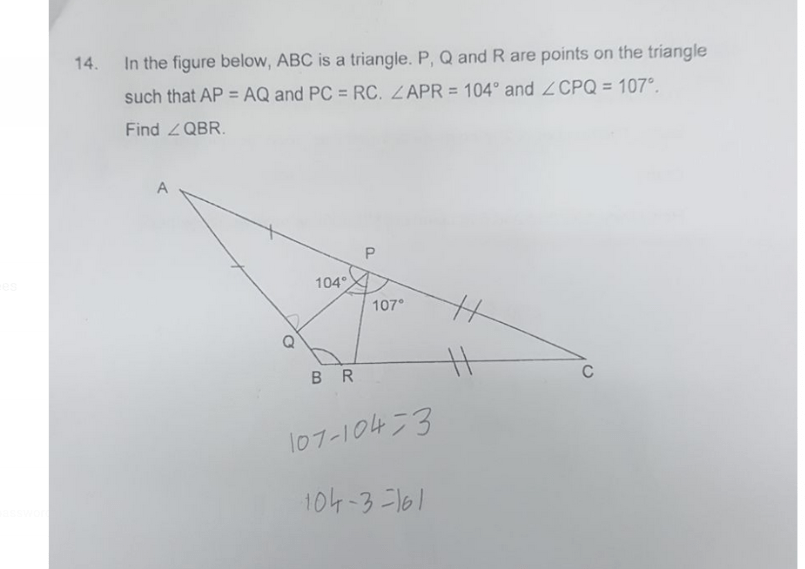# Questionpl. help this p6 home problem

Method 1 :
angle QPR = 104 + 107 – 180 = 31 (sum of angles on a str. line)
angle AQP = angle APQ = 104 – 31 = 73 degrees (isos. triangle)
angle CRP = angle CPR = 107 – 31 = 76 degrees (isos. triangle)
angle PQB = 180 – 73 = 107 (sum of angles on a str. line)
angle PRB = 180 – 76 = 104 (sum of angles on a str. line)
angle QBR = 360 – 104 – 107 – 31 = 118

Method 2 :
angle QPR = 104 + 107 – 180 = 31 (sum of angles on a str. line)
angle APQ = angle AQP = 104 – 31 = 73 degrees (isos. triangle)
angle PAQ = 180 – 2 x 73 = 34 (isos. triangle)
angle CPR = angle CRP = 107 – 31 = 76 degrees (isos. triangle)
angle PCR = 180 – 2 x 76 = 28 (isos. triangle)
angle QBR = 180 – 34 – 28 = 118

Ans : 118 degrees.

0 Replies 0 Likes